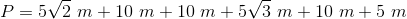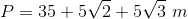# High School Math : How to find the perimeter of a trapezoid

## Example Questions

### Example Question #1 : How To Find The Perimeter Of A Trapezoid

This figure is an isosceles trapezoid with bases of 6 in and 18 in and a side of 10 in.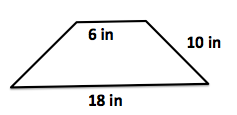What is the perimeter of the isoceles trapezoid (in.)?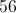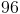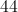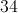Explanation:

The perimeter of the isoceles trapezoid is the sum of all the sides.  You can assume the left side is also 10 in. because it is an isoceles trapezoid.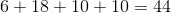### Example Question #2 : How To Find The Perimeter Of A Trapezoid

Find the perimeter of the following trapezoid: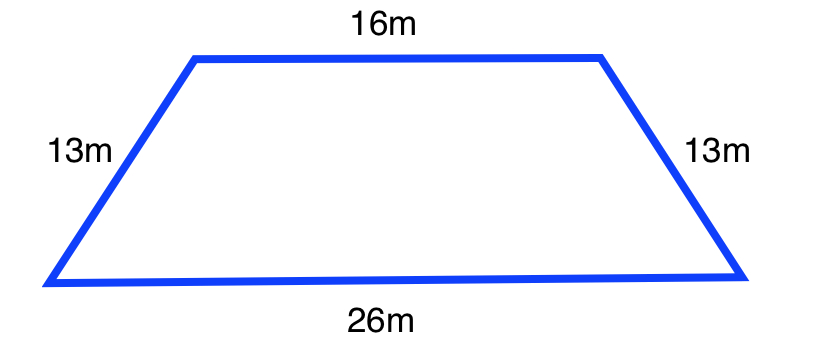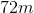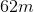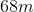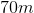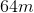Explanation:

The formula for the perimeter of a trapezoid is: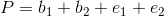Whereis the base and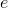is the edge

Plugging in our values, we get: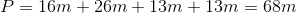### Example Question #3 : How To Find The Perimeter Of A Trapezoid

Find the perimeter of the following trapezoid: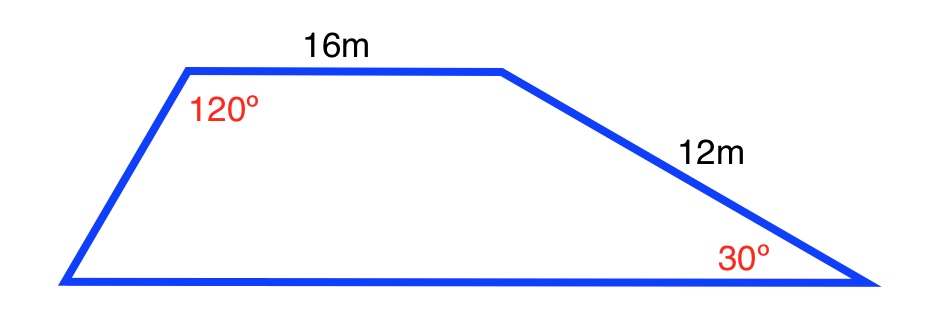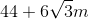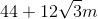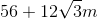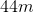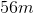Explanation:

Use the formula for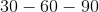triangles in order to find the lengths of all the sides and bases.

The formula is: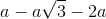Whereis the length of the side opposite the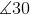.

Beginning with the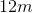side, if we were to create atriangle, the length of the base is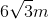, and the height is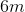.

Creating anothertriangle on the left, we find the height is, the length of the base is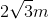, and the side is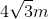.

The formula for the perimeter of a trapezoid is:Whereis the base andis the edge

Plugging in our values, we get: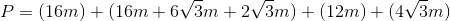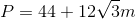### Example Question #4 : How To Find The Perimeter Of A Trapezoid

Determine the perimeter of the following trapezoid: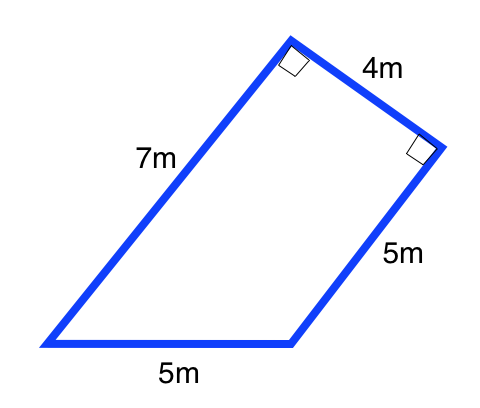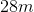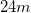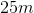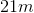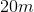Explanation:

The formula for the perimeter of a trapezoid is:,

whereis the length of the base andis the length of the edge.

Plugging in our values, we get: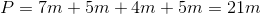### Example Question #5 : How To Find The Perimeter Of A Trapezoid

Find the perimeter of the following trapezoid: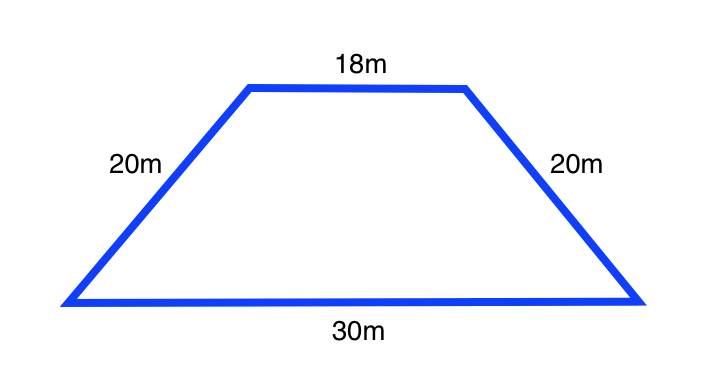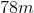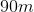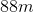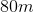Explanation:

The formula for the perimeter of a trapezoid is:,

whereis the length of the base andis the length of the edge.

Plugging in our values, we get: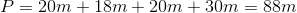### Example Question #6 : How To Find The Perimeter Of A Trapezoid

Find the perimeter of the following trapezoid: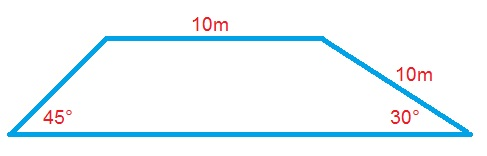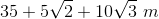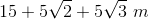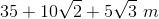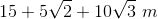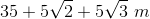Explanation:

The formula for the perimeter of a trapezoid is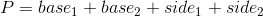.

Use the formula for atriangle to find the length of the base and side: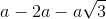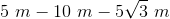Use the formula for a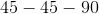triangle to find the length of the base and side: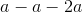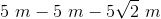Plugging in our values, we get: